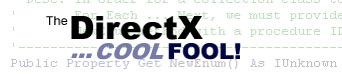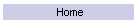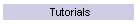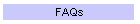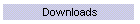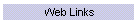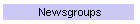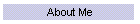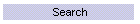## Article

View Oriented Billboards

Microsoft MVP: Eric DeBrosse
This is a direct adaptation of an original C++ article by Robert Dunlop.
Content may not be duplicated without permission.
Updated: 2-7-2002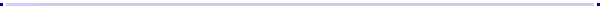### Introduction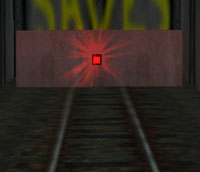Billboarding techniques are utilized in 3D applications to orient a polygon to always face the user.  This allows the application of a 2D image, such as a lens flare or images of foliage, into a 3D scene.  By applying an image in this way, the illusion of complex objects or effects can be applied with a minimum of overhead.

### Viewing a Matrix as a Group of Vectors

To understand the method that we will use here, we need to take an alternate look at our 4x4 viewing matrix.  Ignoring the fourth column, we can divide a matrix into four vectors:

 Rotation Vectors Translation Vector Right Vector Up Vector Forward Vector M14 M24 M34 M44 M11 M21 M31 M12 M22 M32 M13 M23 M33 M41 M42 M43

With this knowledge in hand, we can calculate the location for each corner of a billboard so that it is perpendicular to the field of view, i.e. facing the viewer.

### Extracting the Vectors

To begin with, we will need to retrieve the current viewing matrix and extract the Right and Up vectors from the matrix.  The vectors will then be normalized, so that we can readily scale them to the desired size.  The vectors will then be scaled to one half of the billboard size, so that they represent a distance from the center of the billboard:

Declarations:

 Global g_oDevice As Direct3DDevice8    'initialized device Private m_Size As Single               'billboard size Private m_Color As Long                'billboard diffuse color Private m_Specular As Long             'billboard specular color Private m_Pos As D3DVECTOR             'billboard position Private m_TempPos As D3DVECTOR Private m_RightVec As D3DVECTOR Private m_UpVec As D3DVECTOR Private m_mat As D3DMATRIX Private m_Verts(4) As D3DLVERTEX       'holds 4 vertices

Extract right and up vectors:

 'get view matrix Call g_oDevice.GetTransform(D3DTS_VIEW, m_mat) 'get right vector With m_RightVec     .x = m_mat.m11     .y = m_mat.m21     .z = m_mat.m31 End With Call D3DXVec3Normalize(m_RightVec, m_RightVec) Call D3DXVec3Scale(m_RightVec, m_RightVec, m_Size * 0.5!) 'get up vector With m_UpVec     .x = m_mat.m12     .y = m_mat.m22     .z = m_mat.m32 End With Call D3DXVec3Normalize(m_UpVec, m_UpVec) Call D3DXVec3Scale(m_UpVec, m_UpVec, m_Size * 0.5!)

### Creating the Billboard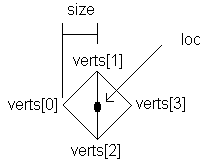All that remains, once we have these vectors, is to create the vertices for the billboard.  In this example, we will use a diamond shaped billboard, which can be rendered as a triangle strip:

Fill vertices:

 'setup each vertex, including color and texture coordinates Call D3DXVec3Subtract(m_TempPos, m_Pos, m_RightVec) m_Verts(0) = MakeD3DLVERTEX(m_TempPos, m_Color, m_Specular, 0!, 0!) Call D3DXVec3Add(m_TempPos, m_Pos, m_UpVec) m_Verts(1) = MakeD3DLVERTEX(m_TempPos, m_Color, m_Specular, 0!, 1!) Call D3DXVec3Subtract(m_TempPos, m_Pos, m_UpVec) m_Verts(2) = MakeD3DLVERTEX(m_TempPos, m_Color, m_Specular, 1!, 0!) Call D3DXVec3Add(m_TempPos, m_Pos, m_RightVec) m_Verts(3) = MakeD3DLVERTEX(m_TempPos, m_Color, m_Specular, 1!, 1!)

Helper function to fill a D3DLVERTEX type:

 Public Function MakeD3DLVERTEX(ByRef Position As D3DVECTOR, _         ByVal lColor As Long, _         ByVal lSpecular As Long, _         ByVal tu As Single, _         ByVal tv As Single) As D3DLVERTEX     With MakeD3DLVERTEX         .x = Position.x         .y = Position.y         .z = Position.z         .Color = lColor         .Specular = lSpecular         .tu = tu         .tv = tv     End With End Function

That's it!  This code will of course have to be repeated every time the view matrix changes, which normally means every frame.  However, the math is pretty light, and the effects that can be added with just two alpha blended polygons is a considerable payoff.

Notes:  Some of the code lines above could be reduced to one line by using simple helper functions.  I have a code module in my downloads section called D3DXHelpers.zip that could be used to return D3DX8 parameters as return values.  For example:

D3DXHelpers.bas Example

 'old way Call D3DXVec3Normalize(m_UpVec, m_UpVec) Call D3DXVec3Scale(m_UpVec, m_UpVec, m_Size * 0.5!) 'new way, with helper functions m_UpVec = Vec3Scale(Vec3Normalize(m_UpVec), m_Size * 0.5!)

Enjoy!

 edebrosse@mvps.org ©2009 Eric DeBrosse www.orionengine.comVisitors since 5/21/2002